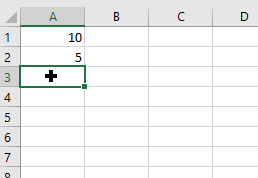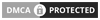You know that the thing of Excel it is one of the biggest most used formulas in a field. Meanwhile if I say that I have a table. In the same way, it contains rows and columns. Of course, column has specific name and rows as well.

Microsoft Excel Courses Online Free in Urdu

We start a formula with two equal signs. For example, I want to use addition operation on spreadsheet I must sell it means that one cell content and value another cell contents. I want to add them both. So that is why I used to apply sum formula of edition. In addition, I call it with equal sign.

What is MS Excel Formulas List with Examples

As a result, we use equal sign in every formula. Okay give the first cell number. Now after that another cell number and finally press enter from my keyboard.

Value Cell1 10 and Cell2 5 Formula =A1+A2 Result 15 See in belowWill help you to understand exactly what I am doing ok so go ahead and check it out below and end this way I am going to tell you what we will cover in our course which is start from basic to advanced level simultaneously.

MS Excel Urdu Tutorials Video Training Course